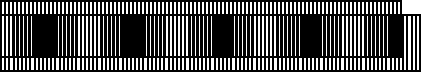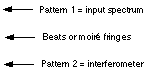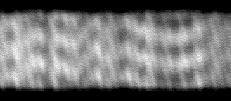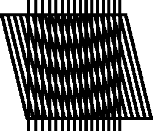Heterodyning creates the moire patternsHeterodyning is a shift in spatial frequencyHeterodyning is the key EDI optical process that allows it to use low resolution spectrographs to measure narrow spectral features. The moire patterns are beats created by the heterodyning process between two different spatial frequencies. One is the input signal and the other is the interferometer transmission function. The latter periodicity is controlled by the interferometer delay, with larger delays making a finer comb (higher spatial frequency). Generally, heterodyning is the shift in frequency f1 of a signal by a fixed amount f2, caused when the input signal is multiplied by a sinusoidal pattern having frequency f2. It is an electrical engineering term-- heterodyning is a processed used in almost all radios, TV's and radars to shift high frequency waves down to lower frequencies where they can be processed by lower cost components. Generally both upshifting (f=f1+f2) and downshifting (f=f1-f2) heterodyning occurs. In the EDI, spectrograph blurring usually obscures the upshifted component so we ignore it (and it can be distinguished from the downshifted signal by the way it responds to phase stepping). The heterodyning process is illustrated in the animation below, where an input spatial frequency of 100 waves per bandwidth is overlayed with one of 96, to creates beats or moire having spatial frequency of 100-96=4 per bandwidth.Stellar spectrum contains a variety of spatial frequenciesThe heterodyning process is illustrated most clearly with a single spatial frequency for the input, but realistic input spectra are rarely so periodic. Instead, they contain a variety of spatial frequencies. Through the process of Fourier decomposition, any arbitrary input signal can be considered to be a sum of many different pure frequency terms with various amplitudes. Even a single absorption line can be thought of this way. Since heterodyning shifts every component the same amount, then if the input spectrum is plotted as a distribution vs spatial frequency, this entire distribution is shifted along the spatial frequency axis.Animation illustrating heterodyning. Remember that any input spectrum can be considered to be a sum of various spatial frequencies of various amplitudes (Fourier decomposition). Here we examine what happens to a single component frequency. The interferometer creates one of the regular patterns, the input signal creates the other. The two patterns have slightly different spatial frequencies, 100 and 96 waves (per distance), creating a beat frequency of 100-96=4. Small horizontal displacement of pattern 1, our pretend input spectrum, creates a very large shift in the moire pattern. Hence the moire is a sensitive measure of small wavelength displacements, such as caused by the Doppler effect.Effect of blurring: the beats persist, even though the underlying patterns which created them are not resolved.Example of heterodyning in actual EDI dataActual (LEFT) and simple graphical simulation (RIGHT) of an iodine spectrum measured by an EDI. Sections of the iodine spectrum have groups of lines that are nearly periodic, with a spacing that slowly changes. This creates a smile-like moire pattern, where the moire phase slowly varies vs wavelength. The moire patterns here are clearly broad, and are therefore of low spatial frequency. Heterodyning shifted the originally high spatial frequency to this low frequency.www.SpectralFringe.org site maintained byDavid Erskineerskine1@llnl.gov Back to EDI home